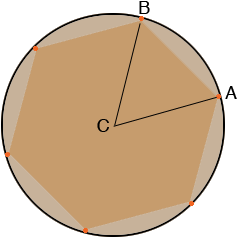SEARCH HOMEMath Central Quandaries & QueriesQuestion from Bobby: What Is The Cord Dimension For 6 Holes On A 24" Bolt CircleHi Bobby,

If I understand your question correctly you have 6 holes equally spaced around the circumference of a 24 inch diameter circle and you want to know the distance between two adjacent holes. In my diagram you want to know the distance from $A$ to $B.$$C$ is the centre of the circle and hence $|CA| = |BC| = 12$ inches and the triangle $ABC$ is isosceles Since there are 6 holes the measure of the angle $BCA$ is $\frac{360}{6} = 60$ degrees. Hence the measures of the angles $ABC$ and $CAB$ are equal and the triangle $ABC$ is equilateral. Thus $|CA| = |AB| = |AC| = 12$ inches.

I hope this helps,
PennyMath Central is supported by the University of Regina and the Imperial Oil Foundation.